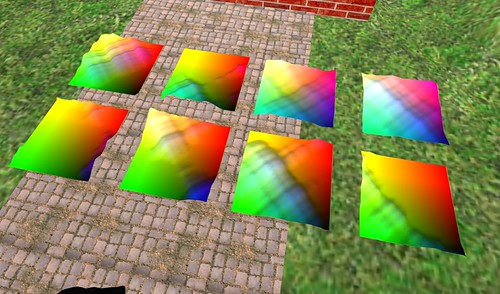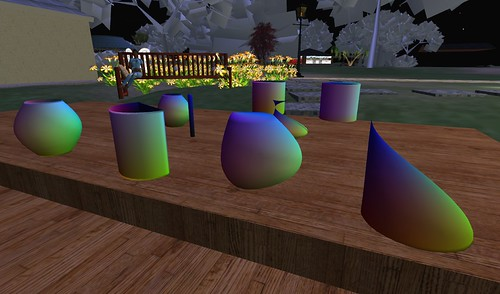## Fractal Heightmap Sculpties

Because I Can. :)This is all the same Python program as before, with the getR and getG functions changed to return simple linear functions of x and y (making the basic plane), and getB changed to return a point from a heightmap, where the heightmap is calculated from an old quasi-self-similar algorithm that I made up years an’ years ago, that produces kinda neat-looking terrains, except that they always have a bias toward diagonal ridges that I’ve been too lazy to squeeze out. Some have a “water table” below which the terrain can’t go, and some don’t.

And you have to set the stitching type to “plane”. I think it is.

In all of these scuplties, btw, I’ve been using the same texture for the visible texture as for the sculpt texture, just to make it more obvious to me what’s going on. That’s not at all required, though; these could just as easily be shiny copper, or grass, or marble, or wood, or what-have-you…

## Making sculpties with code

I finally got around to doing this!Here I am sitting in my place in Inworldz (where the texture uploads are free, heh heh), trying out the sculpt-maps made by this Python program that I wrote that makes sculpt maps.

My favorite so far is the pointy one on the right there, which resulted from a couple of bugs in the program. :)

(And yeah, the Linden trees are being mysteriously grey again.)

Here are a couple of the sculpt textures themselves:

The fun pointy one:Another odd bug-generated one:And the very symmetrical one on the left, once all the bugs were out:And finally (Secrets of the Ages Revealed!) the Python code itself:

```import sys
import math
from PIL import Image

X = 64
Y = X

fname = sys.argv if len(sys.argv)>1 else "sculpt.png"

def getR(x,y):
return(      math.sin( y * 2 * math.pi )  *
(( math.sin( x * math.pi ) + 1 ) / 2)         )

def getG(x,y):
return(      math.cos( y * 2 * math.pi )  *
(( math.sin( x * math.pi ) + 1 ) / 2)        )

def getB(x,y):
return( x*2 - 1  )

def gogogo():
ary = []
for x in range(X):
for y in range(Y):
ary.append( (
127+int(127.0* getR(float(x)/X,float(y)/Y)),
127+int(127.0* getG(float(x)/X,float(y)/Y)),
127+int(127.0* getB(float(x)/X,float(y)/Y))  ) )
mode = "RGB"
size = (X,Y)
im = Image.new(mode,size)
im.putdata(ary)
im.save(fname,"PNG")

gogogo()```

I trust it’s obvious how to use it.

Hahaha, sorry! Couldn’t resist. :)

To use it, well, first install Python and also the Python Imaging Library, then create a “mksc.py” or whatever you want to call it from the code above. Do “python mksc.py vase.png”, and it will create a file “vase.png” which you can upload and use as a sculpt texture to make the symmetrical thing on the left.

Then edit your mksc.py to change the getR, getG, and getB functions to something else, and do “python mksc.py somethingelse.png”, and upload somethingelse.png and use it as a sculpt texture, and see what happens!

For best results, getR, getG, and getB should all expect to get two floating point numbers between zero and one as input, and should return a single floating point number between negative one and one as output.

And there ya go! :)

Oh, and: you may find that the textures work best if uploaded with lossless compression (probably a checkbox on your image-upload panel), and that it’s more obvious what’s going on it you use the “torus” stitching type (in the edit panel right under where you put the sculpt texture) rather than the default “sphere” type. P’haps…